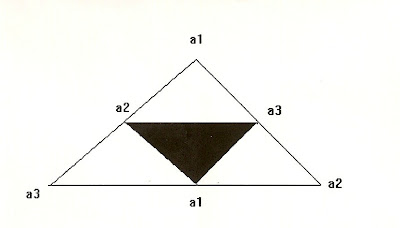## Tuesday, September 26, 2017

### Math Revisited: FractalsFig. 1 - Base space gasketFig. 2: A  2-space gasket

We return to fractals again, this time to examine them in more detail at a basic level. Here I want to compare two simple fractal spaces based on an object known as "the Sierpinski gasket". The most elemental form is depicted in Fig. 1 above and can be compared with Fig 2 which is generated from it.

For Fig. 1, we regard the simple (1/a) space as the generator of more complex fractal spaces F that are essentially infinite. To undertake the cyclic generation process we require that the specification of the vertices (a1, a2, a3) be non-degenerate, i.e. no more than one eigenvalue: a1, a2, or a3 can be assigned for any triangular space. The fundamental space depicted in Fig. 1 we shall call a "Planckian gasket" and note that its fractal mass density can be found as well as its fractal dimension.

The fractal mass density can be computed from:

r (f) = {N(s) - N(h)}/ N(s)

or the number of scale elements minus "hole" elements divided by scale elements.

In this case: N(s) = 1, and N(h) = 0, so:

r (f) = {1 - 0}/ 1 = 1

The fractal dimension D f is the inverse, or: D f = 1/ r (f)

In this case,  D f = 1

Now, examining the larger space (2-space)  in Fig. 2 defined by {2/a}, we again obtain the vertex designation (a1, a2, a3).

However, we further note that to get from the primitive space to the space {2/a} we require the transposition (see the blog entry on transpositions from two years ago):

(a1, a2, a3) -> (a1, a3, a2)

That is, a1 remains fixed, and a2 - a3 are "mirrored" through a bisector axis identified above from, e.g.  a1-a1. Note that the "hole" (in black) represents the inversion space (or negative space: -a1a2a3) a result of the inversion of the positive space of the primitive {1/a}.

The fractal mass density can be computed from:

r (f)  ={4 - 1}/ 4 = 3/4 = 0.75

And the fractal dimension is:

D f = 1/ r (f) = 1/ 0.75 = 1.333

Exercise for the math-minded reader: Draw the space for {3/a} with three symmetric holes of oriented vertices a1, a2, and a3 inside it. Then work out the fractal mass density and fractal dimension.